# Setup R Envionment

If you have never used the aqp or soilDB packages before, you will likely need to install them. This only needs to be done once.

``````# stable versions + deps
install.packages('aqp', dep=TRUE)
install.packages('soilDB', dep=TRUE)
install.packages('sharpshootR', dep=TRUE)

Once you have all of the R packages on which this document depends, it is a good idea to load them. R packages must be installed anytime you change versions of R (e.g., after an upgrade) and loaded anytime you want to access functions from within those packages.

``````library(aqp)
library(soilDB)
library(sharpshootR)
library(cluster)
library(RColorBrewer)
library(ape)``````

# Simple Example via Soil Texture

``````# soil textures, sorted according to field book
data("ROSETTA.centroids")

# ordered vs. nominal texture classes
tex.ordered <- ROSETTA.centroids\$texture
tex.nominal <- factor(ROSETTA.centroids\$texture, levels=sort(as.character(ROSETTA.centroids\$texture)), ordered = FALSE)

# assemble into DF, note that stringsAsFactors=FALSE required
x.1 <- data.frame(
p1=tex.ordered,
stringsAsFactors = FALSE
)

x.2 <- data.frame(
p1=tex.nominal,
stringsAsFactors = FALSE
)

# copy texture classes to row names for automatic distance matrix / clustering labels
row.names(x.1) <- x.1\$p1
row.names(x.2) <- x.2\$p1

# 1D clustering
d.1 <- daisy(x.1[, 1, drop=FALSE], metric = 'gower')
d.2 <- daisy(x.2[, 1, drop=FALSE], metric = 'gower')

## note differences
# d.1
# d.2

# check: pair-wise distances should reflect information contained in ranks of ordinal representation
par(mar=c(1,1,3,1), mfcol=c(1,2))
plot(as.phylo(as.hclust(diana(d.1))), main='Ordinal', label.offset=0.01)
plot(as.phylo(as.hclust(diana(d.2))), main='Nominal', label.offset=0.01)``````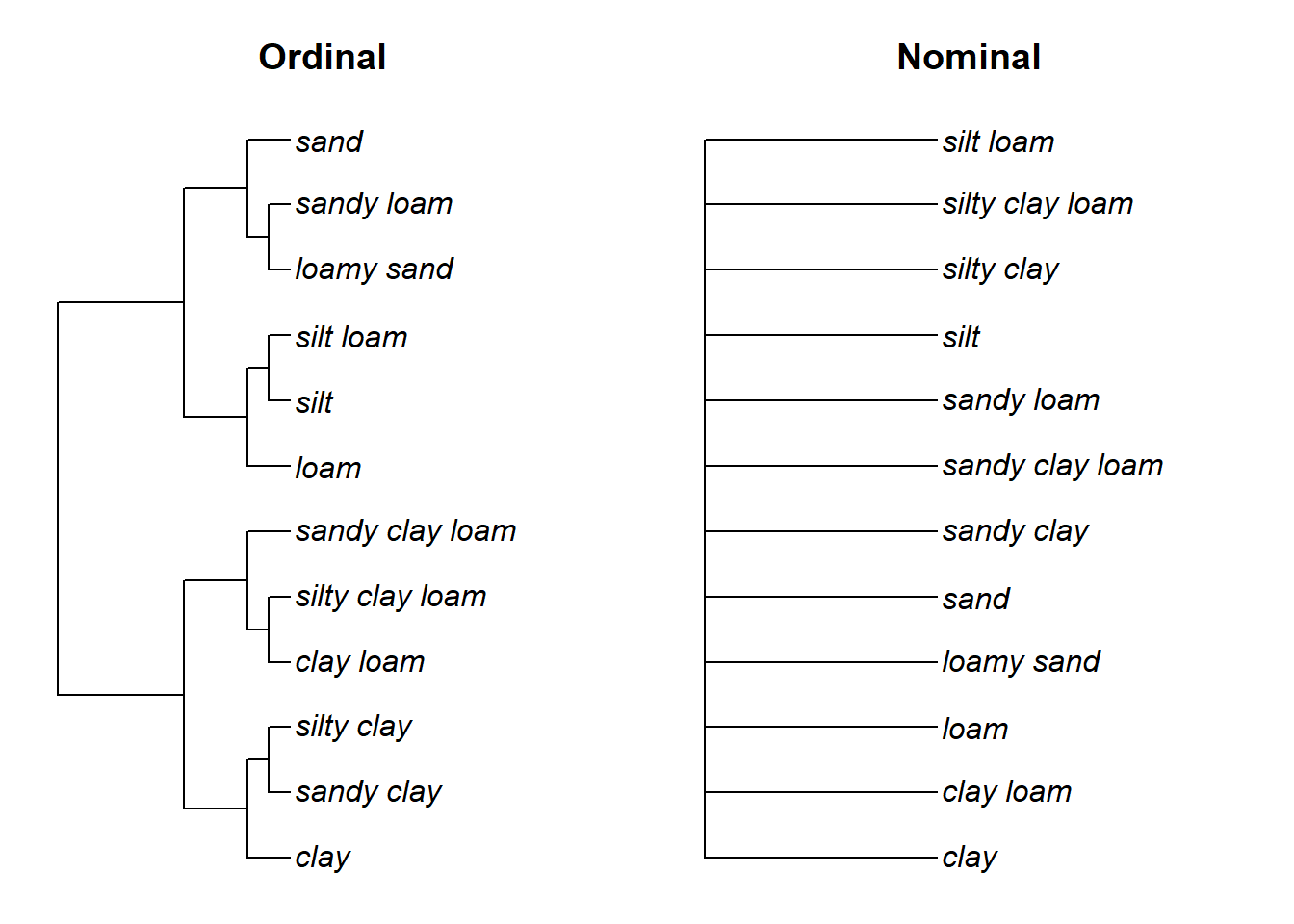Another reason to use ordered factors when possible: cophenetic correlation.

``````# ordinal
cor(d.1, cophenetic(as.hclust(diana(d.1))))``````
``##  0.7105597``
``````# nominal
cor(d.2, cophenetic(as.hclust(diana(d.2))))``````
``## Warning in cor(d.2, cophenetic(as.hclust(diana(d.2)))): the standard deviation is zero``
``##  NA``

# Sample Data

While the methods outlined in this document can be applied to any collection of pedons, it is convenient to work with a standardized set of data. You can follow along with the analysis by copying code from the following blocks and running it in your R session. The sample data used in this document is based on soil profiles that have been correlated to the Loafercreek soil series from the Sierra Nevada Foothill Region of California. Note that the internal structure of the `loafercreek` data is identical to the structure returned by `fetchNASIS()` from the soilDB package. All horizon-level values are pulled from the pedon horizon table of the pedons being analyzed.

``````# load sample data from the soilDB package
data(loafercreek, package = 'soilDB')

# get a subset of profiles to work with
x <- loafercreek[10:20, ]``````

# Methods

## Check Data

``````# check existing textures
table(x\$texture_class)``````
``````##
##  br   c  cb  cl  gr   l scl sil  sl
##   9   4   1  13   2  32   1   5   2``````
``````# order field texture according to particle size
# note: leaving out "in-lieu-of" texture classes for organic soil material and bedrock
x\$texture_class <- factor(x\$texture_class, ordered = TRUE, levels = SoilTextureLevels())
x\$texture_class <- droplevels(x\$texture_class)

# make a copy without ordering
x\$texture_class_nominal <- factor(x\$texture_class, levels=sort(as.character(unique(x\$texture_class)), decreasing = TRUE), ordered = FALSE)

# graphical check
par(mar=c(0,1,3,1))
plot(x, color='texture_class', label='pedon_id')``````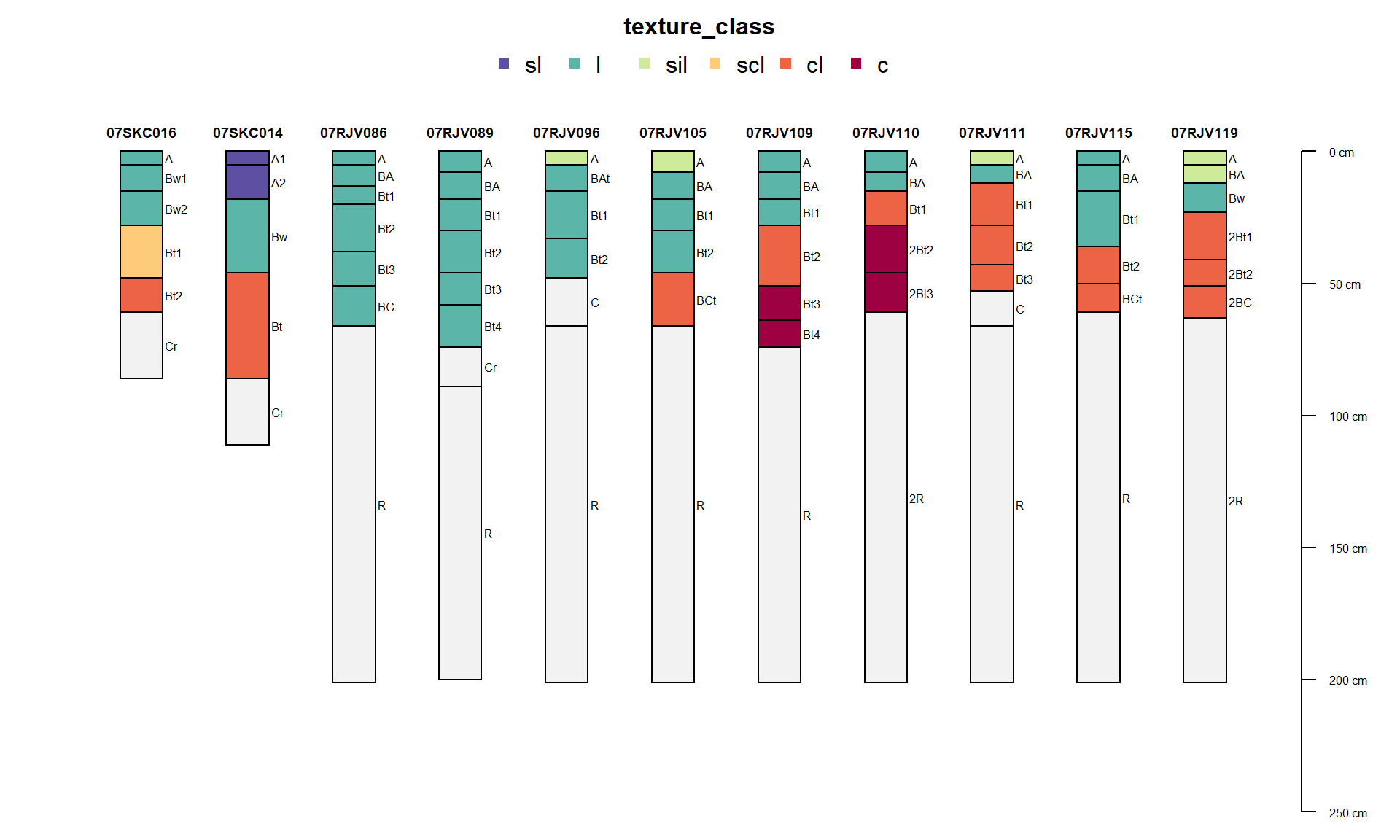``plot(x, color='texture_class_nominal', label='pedon_id')``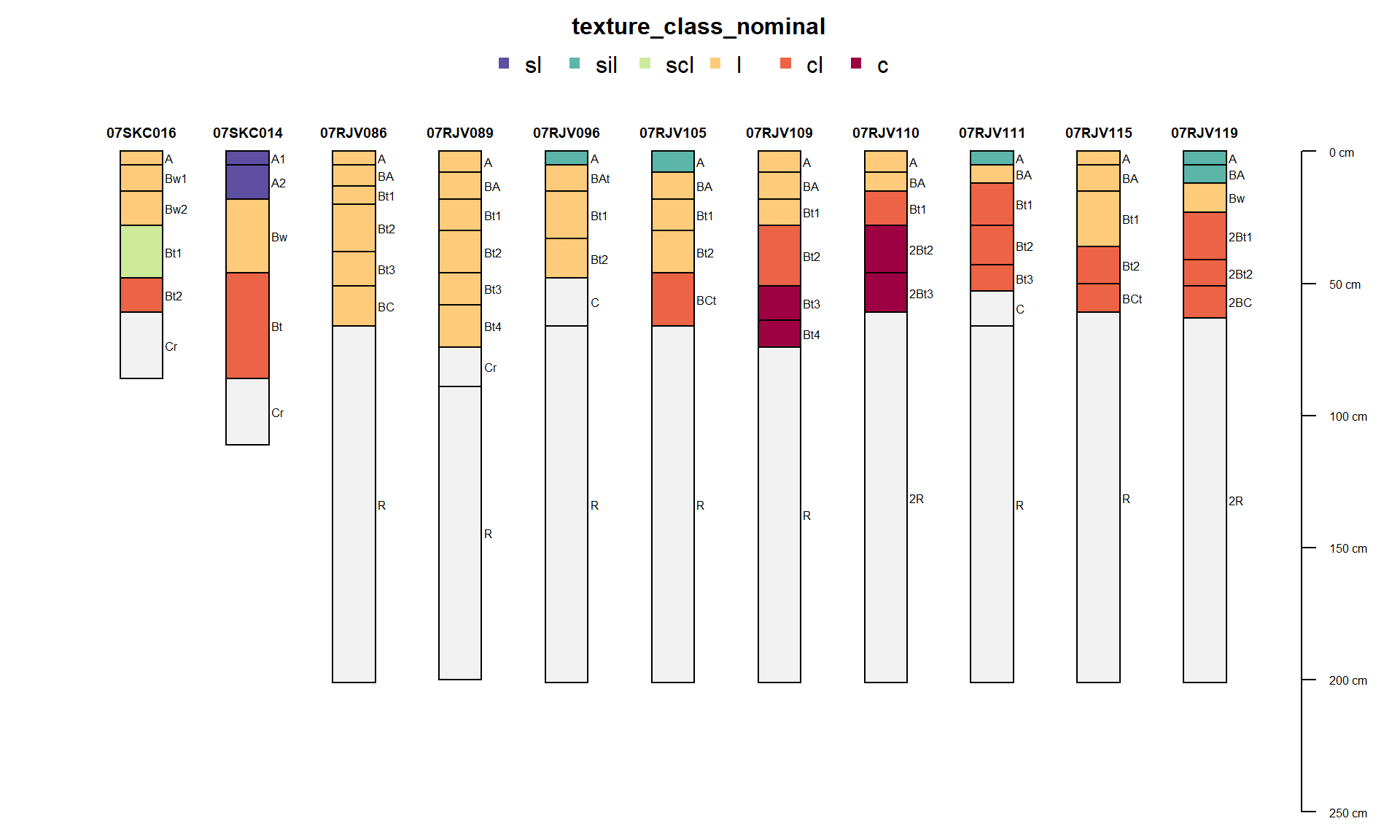``````# make a fake horizon-level attr, until #7 is fixed
x\$constant <- rep(1, times=nrow(x))``````

## Horizon Level Attributes

``````# compute pair-wise distances using texture class (ordered factor)
d.hz <- profile_compare(x, vars=c('texture_class', 'constant'), max_d=100, k=0, rescale.result=TRUE)
# divisive hierarchical clustering
dd.hz <- diana(d.hz)

# plot dendrogram + profiles
par(mar=c(1,0,3,1))
plotProfileDendrogram(x, dd.hz,  width=0.25, color='texture_class', label='pedon_id', cex.name=0.65)``````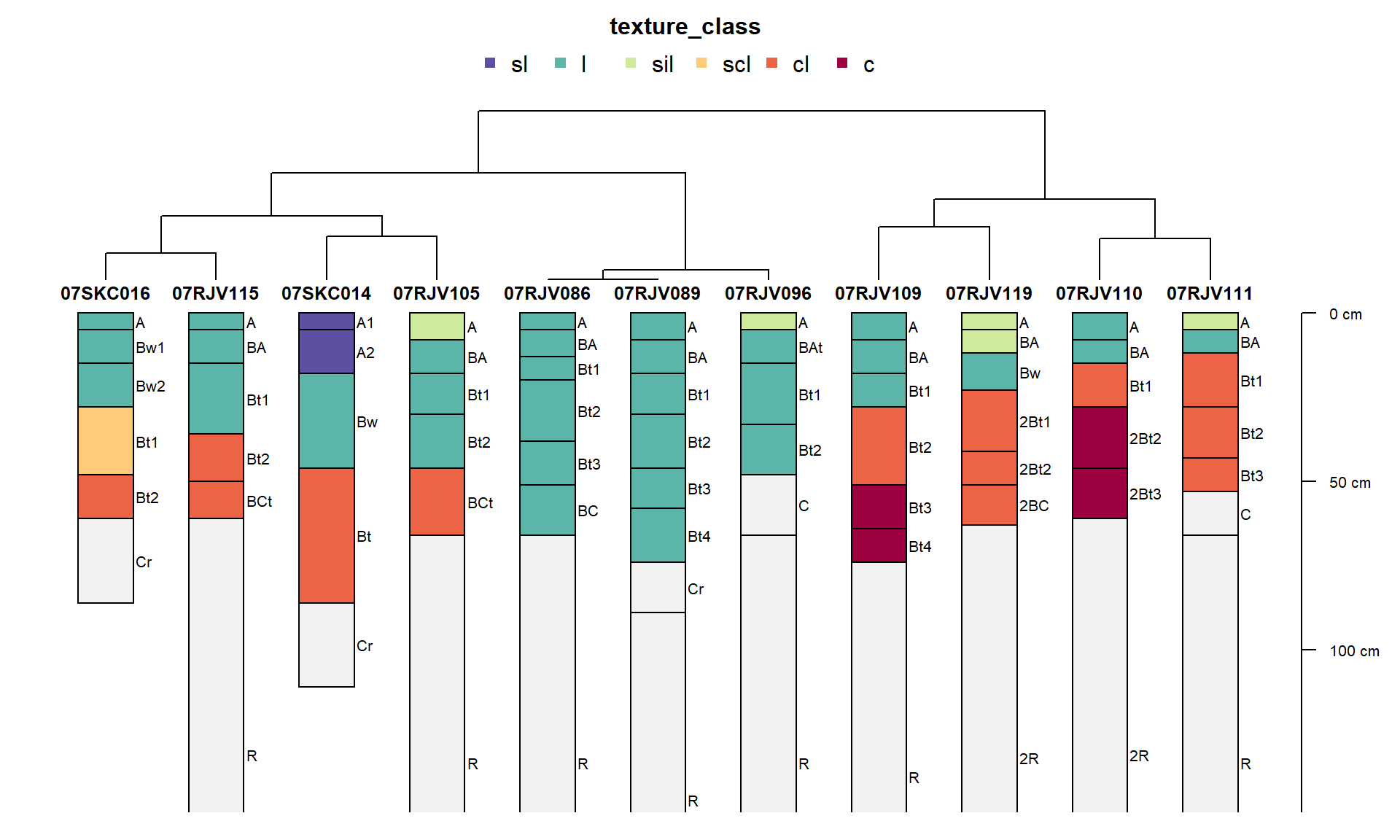``````# compute pair-wise distances using texture class (nominal factor)
d.hz <- profile_compare(x, vars=c('texture_class_nominal', 'constant'), max_d=100, k=0, rescale.result=TRUE)
# divisive hierarchical clustering
dd.hz <- diana(d.hz)

# plot dendrogram + profiles
par(mar=c(1,0,3,1))
plotProfileDendrogram(x, dd.hz,  width=0.25, color='texture_class_nominal', label='pedon_id', cex.name=0.65)``````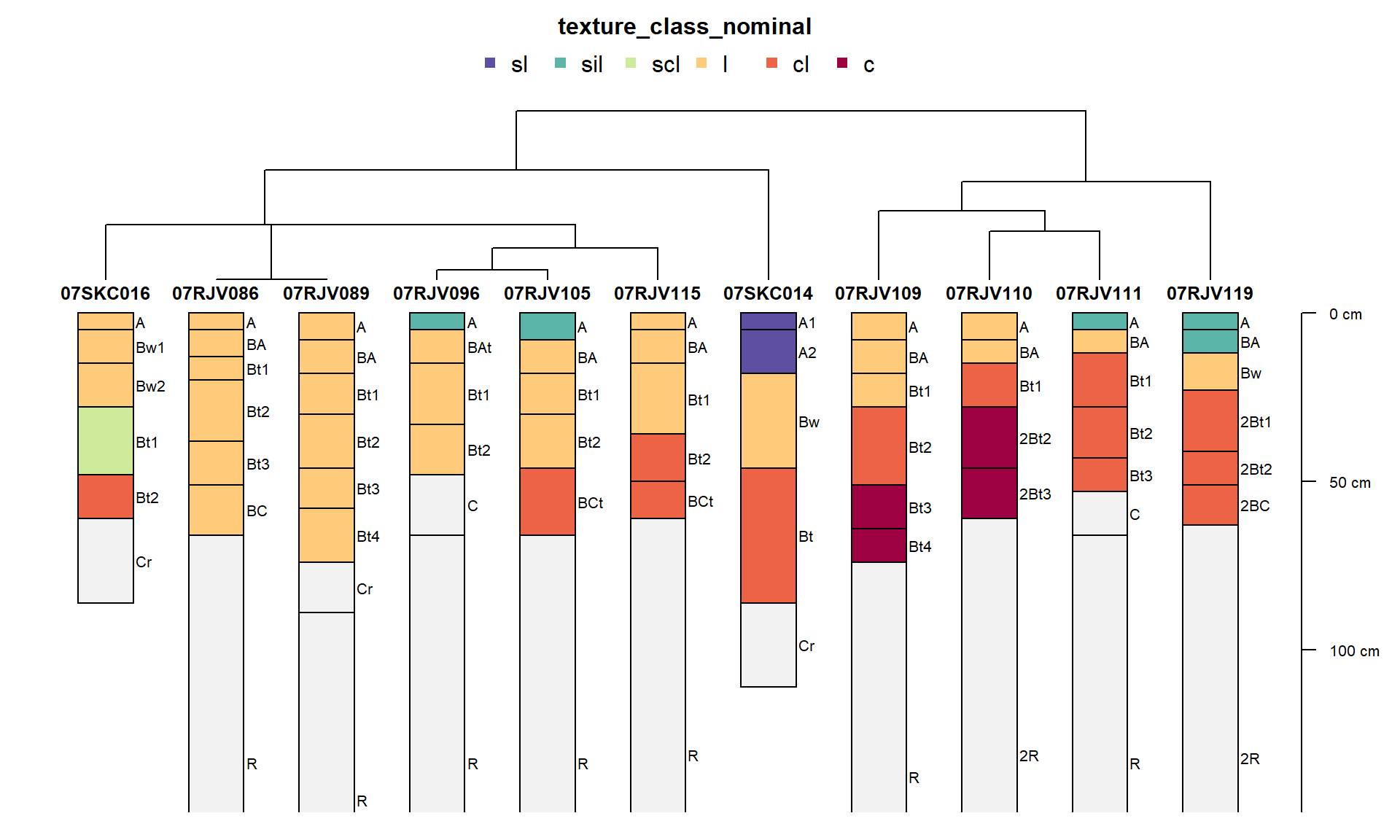### Compare Dendrograms

``````d.ordinal <- profile_compare(x, vars=c('texture_class', 'constant'), max_d=100, k=0, rescale.result=TRUE)

d.nominal <- profile_compare(x, vars=c('texture_class_nominal', 'constant'), max_d=100, k=0, rescale.result=TRUE)

p.ordinal <- as.phylo(as.hclust(diana(d.ordinal)))
p.nominal <- as.phylo(as.hclust(diana(d.nominal)))

dueling.dendrograms(p.1 = p.ordinal, p.2 = p.nominal, lab.1 = 'Ordinal', lab.2 = 'Nominal')``````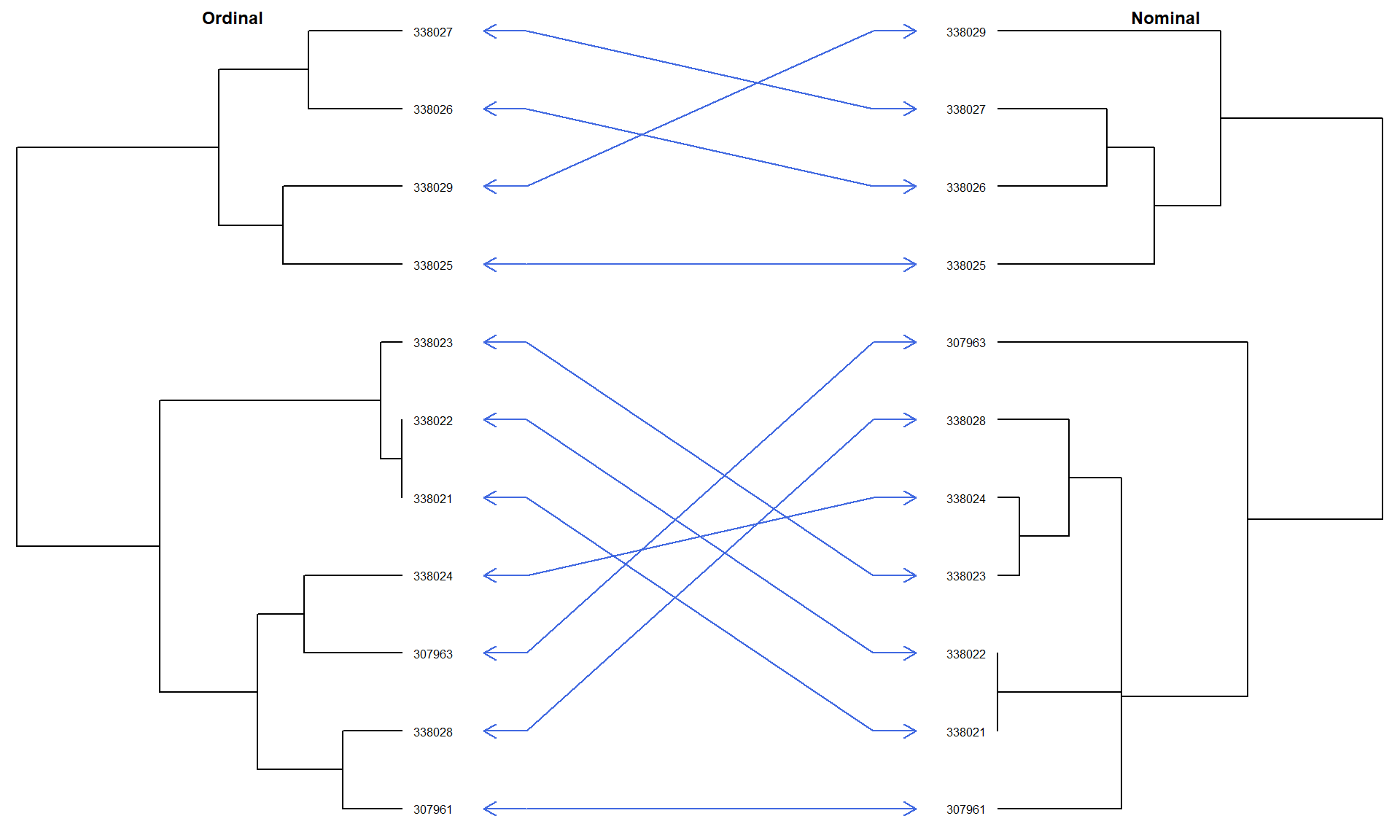``````# dendextend::dend_diff(as.dendrogram(diana(d.ordinal)), as.dendrogram(diana(d.nominal)))

# dendextend::tanglegram(as.dendrogram(diana(d.ordinal)), as.dendrogram(diana(d.nominal)))``````

## Site and Horizon Level Attributes

``````# fix some missing data
x\$elev_field[x\$elev_field == 0] <- 380

# graphical check: order pedons by slope
par(mar=c(0,1,3,1))
plot(x, color='texture_class', label='pedon_id', col.palette=brewer.pal(10, 'Spectral'), plot.order=order(x\$slope_field))
axis(side=1, at=1:length(x), labels=x\$slope_field[order(x\$slope_field)], line=-3)
mtext(text='Field Described Slope (%)', side=1, line=-4)``````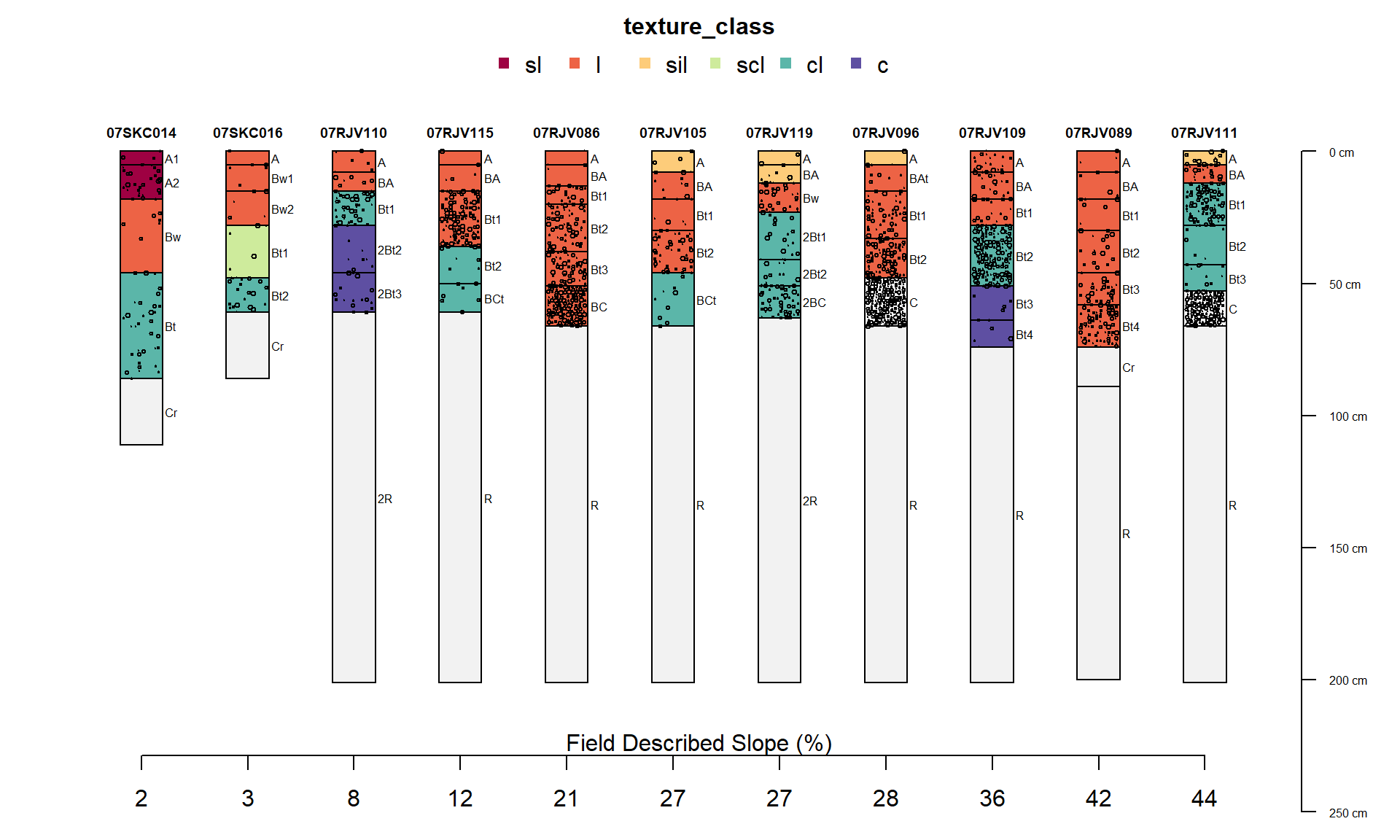``````# graphical check: order pedons by elevation
par(mar=c(0,1,3,1))
plot(x, color='texture_class', label='pedon_id', col.palette=brewer.pal(10, 'Spectral'), plot.order=order(x\$elev_field))
axis(side=1, at=1:length(x), labels=x\$elev_field[order(x\$elev_field)], line=-3)
mtext(text='Field Described Slope (m)', side=1, line=-4)``````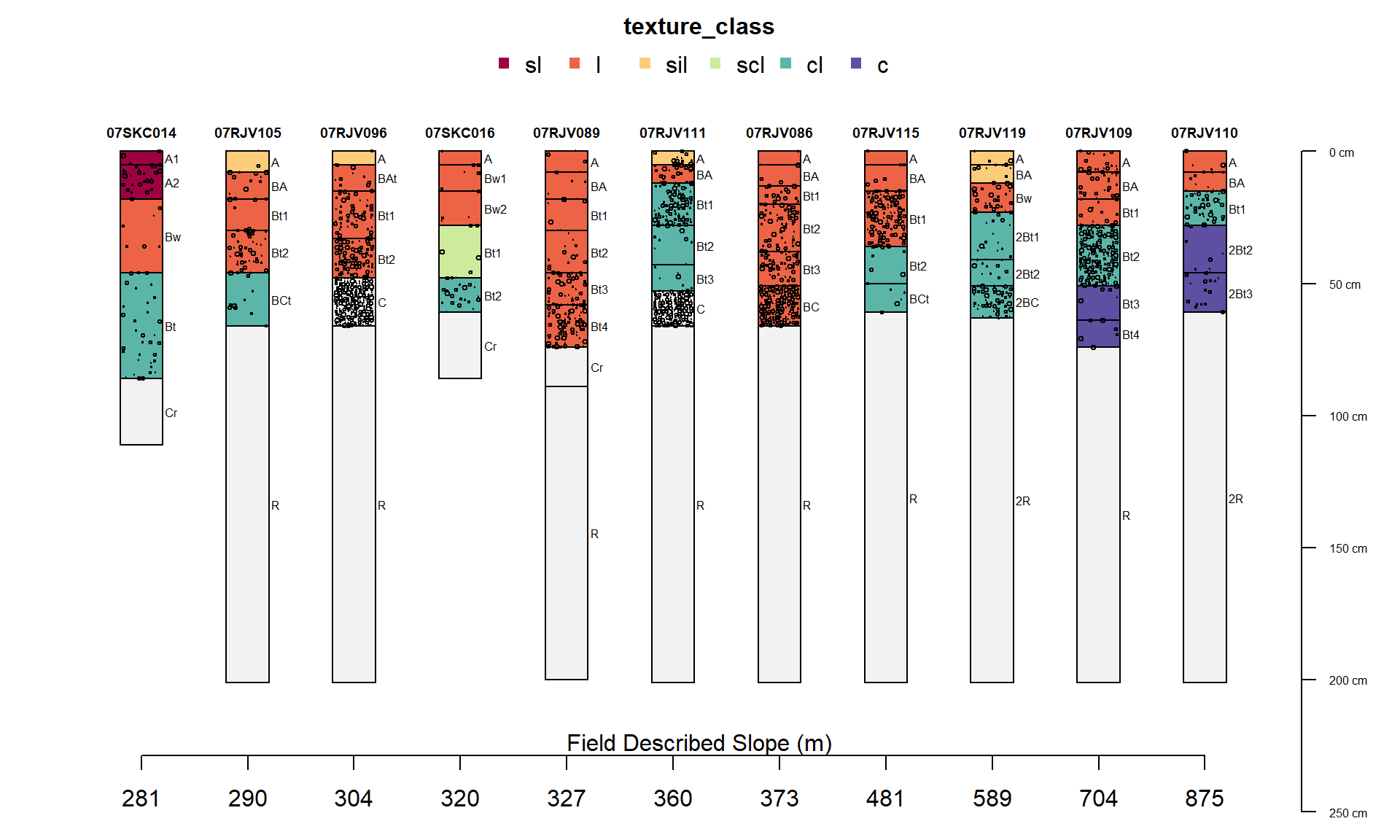``````# compute pair-wise distances using:
# hz attributes: texture class (ordered factor) and rock frag volume
# site attributes: surface slope and elevation
d.hz.site <- profile_compare(x, vars=c('texture_class', 'total_frags_pct', 'slope_field', 'elev_field'), max_d=100, k=0)
# divisive hierarchical clustering
dd.hz.site <- diana(d.hz.site)

# plot dendrogram + profiles
par(mar=c(2,1,3,1))
plotProfileDendrogram(x, dd.hz.site, scaling.factor = 0.008, y.offset = 0.1, width=0.15, color='texture_class', name='fake.name', label='pedon_id', cex.name=0.75, col.palette=brewer.pal(10, 'Spectral'))